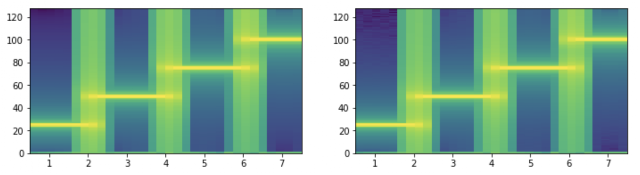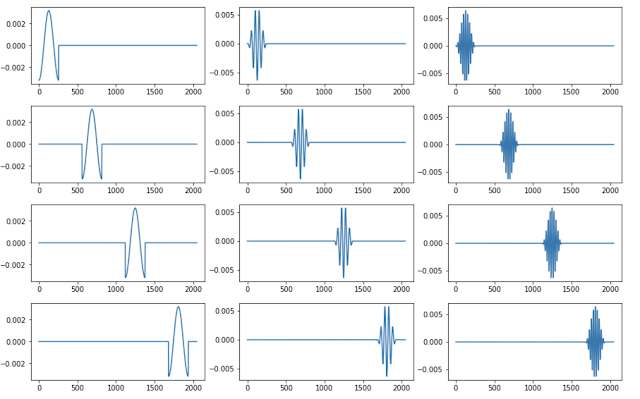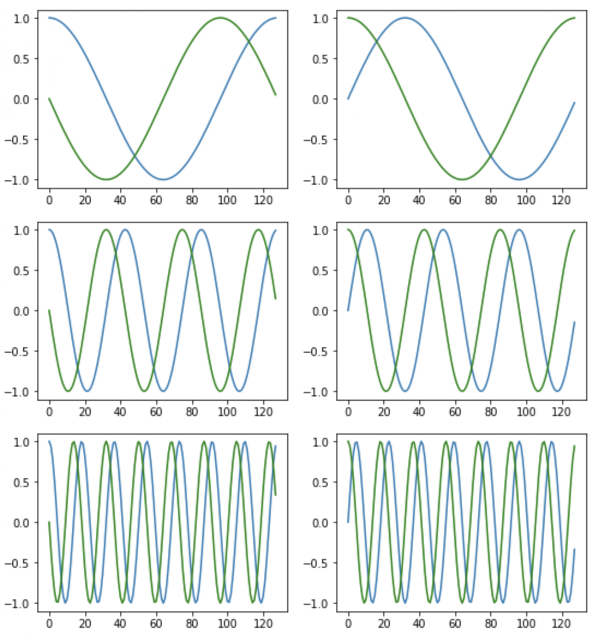Monday, March 02, 2020

Generating Spectrograms with Neural Networks

In a previous experiments, I used spectrograms instead of raw audio as inputs to neural networks, while training them to recognize pitches, intervals, and chords.

I found that feeding the networks raw audio data got nowhere. Training was extremely slow, and losses seemed to be bounded at unacceptably high values. After switching to spectrograms, the networks started learning almost immediately -- it was quite remarkable!

This post is about generating spectrograms with neural networks.These spectrograms were generated by a Neural Network

On Spectrograms

Spectrograms are 2-dimensional visual representations of slices of audio (or really, any signal.) On the x-axis of a spectrogram is time, and on the y-axis is frequency.A Violin playing A4 (440hz)

Because the data is correlated well along both dimensions, spectrograms lend themselves well to both human analysis and convolutional neural networks.

So, I wondered, why can't the networks learn the spectrograms themselves? Under the covers, spectrograms are built with STFTs, which are entirely linear operations on data -- you slide a window over the data at some stride length, then perform a discrete Fourier transform to get the frequency components of the window.

Since the transformation is entirely linear, all you need is one network layer, no activations, no biases. This should theoretically collapse down to a simple regression problem. Right? Let's find out.

Generating Training Data

We start by synthesizing some training data. To keep things simple, let's assume that we want to generate spectrograms of 25ms of audio sampled at 8khz, which is 2000 samples. Round up (in binary) to 2048 to make things GPU friendly.

The underlying STFT will use a hanning window of size 256, FFT size of 256, and a stride length of 250, producing 33x129 images. That's 33 frequency-domain slices (along the time axis) capped at 128hz.

Note that the spectrograms return complex values. We want to make sure the networks can learn to completely reconstruct both the magnitude and phase portions of the signal. Also note that we're going to teach our network how to compute hanning windows.

Here's the code to generate the training data -- we calculate batch_size (15,000) examples, each with 2048 samples and assign them to xs. We then calculate their spectrograms and assign them to ys (the targets.)

Note that we separate the real and imaginary components of the spectrogram and simply stack one atop the other. We also don't scale or normalize the data in any way. Let the network figure all that out! :-)

Building the Model

Now the fun part. We build a single-layer network with 2048 inputs for the audio slice, and row * col outputs for the image (times two to hold the real and imaginary components of the outputs.) Since the outputs are strictly a linear function of the inputs, we don't need a bias term or activation functions.

Again, this is really just linear regression. With, oh, about 17 million variables!

This model trains very fast. In 4 epochs (about 80 seconds), the loss drops to 3.0e-08, which is sufficient for our experiments, and in 10 epochs (about 7 minutes), we can drop it all the way to 2.0e-15.

The Real Test

Our model is ready. Let's see how well this does on unseen data. We generate a slice of audio playing four tones, and compare scipy's spectrogram function with our neural network.Left: SciPy, Right: Neural Network

Wow, that's actually pretty good, however when we look at a log-scaled version, you can see noise in the network-generated one.Log-scaled spectrogram: Left: SciPy, Right: Neural Network

Maybe we can train it for a bit longer and try again.Left: SciPy, Right: Neural Network

Oh yeah, that's way better!

Peeking into the Model

Okay, so we know that this works pretty well. It's worth taking a little time to dig in and see what exactly it learned. The best way to do this is by slicing through the layers and examining the weight matrices.

Lucky for us there's just one layer with (2048, 8514) weights. The second dimension (8154) is just the flattened spectrogram for each sample in the first. In the code above, we reshaped and transformed the data to make it easy to visualize.

Here it is below -- the weight maps for the first, 11th, 21st, and 31st slices (out of 33) of the output.

The vertical bands represent activated neurons. You can see how the bands move from left to right as they work on a 256-sample slice of the audio. But more interesting is the spiral pattern of the windows. What's going on there? Let's slice through one of the bands and plot just the inner dimension.

This is actually pretty cool -- each of the graphs below is a Hanning-Windowed sine wave of an integer frequency along each of the vertical bands. These sinusoids are correlated with the audio, one-by-one, to tease out the active frequencies in the slice of audio.1, 5, and 10hz Sine Waves (Windowed)

To put it simply, those pretty spirally vertical bands are... Fourier Transforms!

Learning the Discrete Fourier Transform

Exploring that network was fun, however we must go deeper. Let's build a quick network to perform a 128-point DFT, without any windowing, and see if there's more we can learn.

This is a much simpler network, with only about 65k weights. It trains very fast, and works like a charm!

Digging into the weights, you can clearly see the complex sinusoids used to calculate the Fourier transform.Real (blue) and Imaginary (green) Components

If you look at the weight matrix as a whole, you see the same pattern we saw in the vertical bands of the spectrogram NN weights.

There's a lot more we can explore in these networks, but I should probably end here... this post is getting way too long.

Final Thoughts

It's impressive how well this works, and how quickly this works. The neural networks we trained above are relatively crude, and there are techniques we can explore to optimize them.

For example, with the spectrogram networks -- instead of having it learn each FFT band independently for each window, we could use a different network architecture (like recurrent networks), or implement some kind of weight sharing strategy across multiple layers.

Either way, let me be clear: using Neural Networks to perform FFTs or generate spectrograms is completely impractical, and you shouldn't do it. Really, don't do it! It is, however, a great way to explore the guts of machine learning models as they learn to perform complicated tasks.